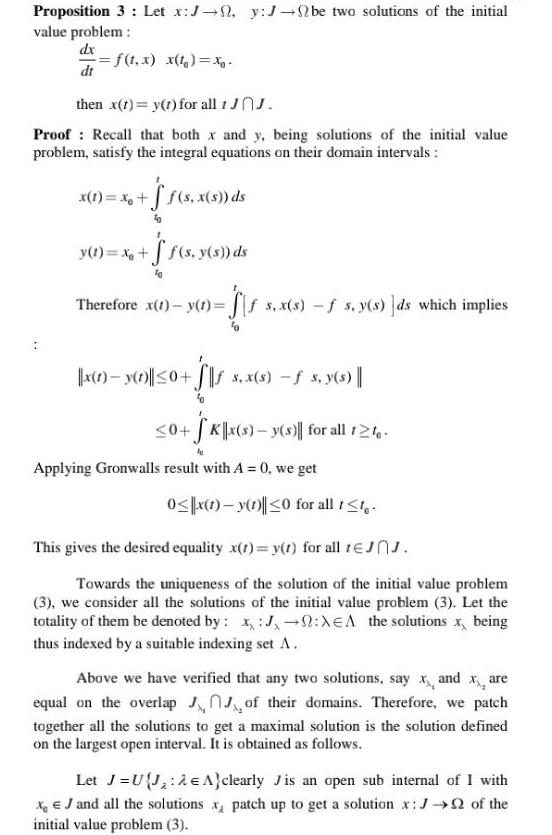Question:

# Proposition 3 Let x J y J be two solutions of the initial

Last updated: 9/18/2023Proposition 3 Let x J y J be two solutions of the initial value problem dx f t x x t x dt then x t y t for all 1 JOJ Proof Recall that both x and y being solutions of the initial value problem satisfy the integral equations on their domain intervals x 1 x f s x s ds y t x f s y s ds Therefore x 1 y 1 f s x s f s y s ds which implies x 1 y 1 0 s x s s y s 0 SK x s y s for all 12 Applying Gronwalls result with A 0 we get 0 x 1 y 1 0 for all t t This gives the desired equality x t y t for all 1JJ Towards the uniqueness of the solution of the initial value problem 3 we consider all the solutions of the initial value problem 3 Let the totality of them be denoted by J XEA the solutions x being thus indexed by a suitable indexing set A Above we have verified that any two solutions say x and xx are equal on the overlap JJ of their domains Therefore we patch together all the solutions to get a maximal solution is the solution defined on the largest open interval It is obtained as follows Let J UJ e clearly Jis an open sub internal of I with x J and all the solutions x patch up to get a solution x J of the initial value problem 3